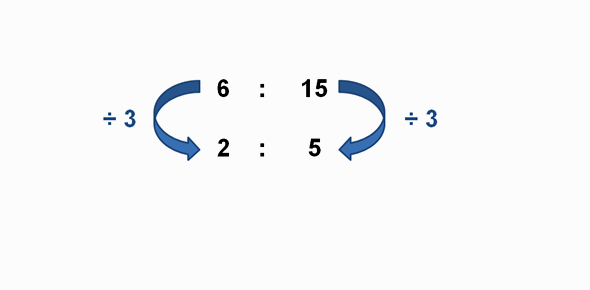What would be the ratio of the radius of the circles to the side of - ProProfs DiscussTopicsMoreProductsMore+ Ask Question

# What would be the ratio of the radius of the circles to the side of the square? Anoop managed to draw 7 circles of equal radii with their centres on the diagonal of a square such that the two extreme circles touch two sides of the square and each middle circle touches two circles on either side.Change Image    Delete

A. 1:(2+ 6√2)
B. 1:(4+ 7√3)
C. (2+ 7√2):1
D. 1:(2+ 7√2)

This question is part of @TCS-mock test
Asked by Mr.venkat, Last updated: Apr 02, 2020

###Request 0FollowShareAnswer AnonymouslyAnswer LaterCopy Link#### John Smith

John Smith1:(2+ 6√2)

The extreme circles will have radius perpendicular to sides of square so the part of diagonal till the centre of circle will be sqrt(2) r and remaining portion is r , 5 more circles will contribute 10r and last circle will contribute r + sqrt(2) r . Length of diagonal = 12r + 2sqrt (2) r = sqrt (2) s where s -> side of square. So, ratio of r:s = 1/( 2 + 6 sqrt(2)) = 1: [ 2+ 6 sqrt(2)]Search for Google imagesSelect a recommended image
Upload from your computerCancelSearch for Google imagesSelect a recommended image
Upload from your computerCancelSearch for Google imagesSelect a recommended image
Upload from your computerCancel